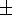Next: Discussion Up: Results Previous: Temporal Structure Measures

### Relationship of Stride Dynamics to Height

In children, many aspects of gait depend on body size. For example, over certain age ranges, stride length increases linearly with age, but the relationship between stride length and age becomes constant after adjusting for height or leg length (2). Similarly, we observed a significant increase (p < .0001) in velocity in the 6 and 7 year old children (1.200.03 m/sec) compared to the 3 and 4 year old children (1.000.03 m/sec). However, relative velocity (velocity/height) was essentially identical in these two groups (p = 0.92). To begin to evaluate whether the changes in stride dynamics were only a function of changes in biomechanics related to growth, we normalized the dynamical measures with respect to height. In the present study, height increased linearly with age (r = 0.96; p < .0001). Variability is inversely related to age (Figure 3). Thus, we normalized the dynamical variables (either by appropriately dividing or multiplying by height) and re-examined the relationship with age. The age-dependence persisted when the measures of variability and dynamics were adjusted for height or if leg length was used instead of height as the normalizing factor. For example, the CV normalized with respect to leg length was 12810, 835, and 673 in the 3 and 4 year olds, 6 and 7 year olds, and in the 11 to 14 year olds, respectively. The difference between the 6 and 7 year olds and the 11 to 14 year olds was significant (p < .05). The CV in the 3 and 4 year old was significantly increased (p < .0005) compared to both older groups.

Finally, we note that consistent with previous findings (26) the average values of stride time and walking velocity were age-dependent. Mean stride time was 90014 msec in the 3 and 4 year olds, increased (p < .01) to 95512 msec in the 6 and 7 year olds, and increased further (p < .0001 ) in the 11 to 14 year olds (107218 msec). Walking velocity was 1.000.03 m/sec in the 3 and 4 year olds, 1.200.03 m/sec in the 6 and 7 year olds, and 1.28.0.03 m/sec in the 11 to 14 year olds. The difference between the youngest and two older groups was significant (p < .0001); however, walking velocity was not significantly different in the 6 and 7 year olds and the 11 to 14 year olds.Next: Discussion Up: Results Previous: Temporal Structure Measures